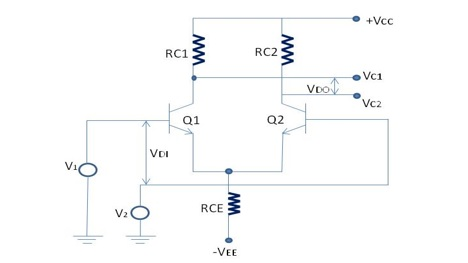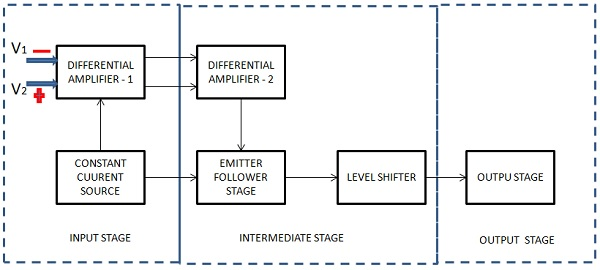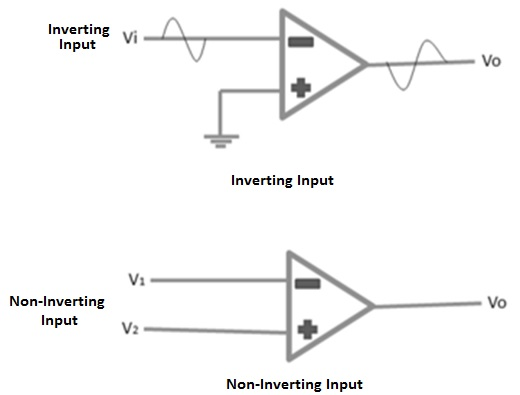# Operational Amplifiers

An operational amplifier, or op-amp, is a very high gain differential amplifier with high input impedance and low output impedance. Operational amplifiers are typically used to provide voltage amplitude changes, oscillators, filter circuits, etc. An op-amp may contain a number of differential amplifier stages to achieve a very high voltage gain.

This is a high gain differential amplifier using direct coupling between the output and the input. This is suitable for DC as well as AC operations. Operational amplifiers perform numerous electronic functions such as instrumentation devices, signal generators, active filters, etc. besides various mathematical operations. This versatile device is also used in many non–linear applications, such as voltage comparators, Analog–to–digital converters and Digital–to–Analog converters, Logarithmic amplifiers, non–linear function generators, etc.

## Basic Differential Amplifier

The following illustration shows a basic differential amplifier −In the above figure −

• VDI = differential input

• VDI = V1 – V2

• VDO = differential output

• VDO = VC1 - VC2

This amplifier amplifies the difference between the two input signals, V1 and V2.

Differential voltage gain,

$$A_d = \frac{V_{DO}}{V_{DI}}$$

and

$$A_d = \frac{(V_{C1} - V_{C2})}{V_{DI}}$$

As shown in the following figure, basic operational amplifier consists of three stages −### Input Stage

This is the first stage and has the following characteristics.

• High CMR (Common Mode Rejection)
• High input impedance
• Wide band width
• Low (DC) input offset

These are some significant characteristics for the performance of the operational amplifier. This stage consists of a differential amplifier stage and a transistor is biased so that it acts as a constant current source. The constant current source greatly increases the CMR of the differential amplifier.

Following are the two inputs to the differential amplifier −

• V1 = Non inverting input
• V2 = Inverting input

### Intermediate Stage

This is the second stage and designed to get better voltage and current gains. The current gain is required to supply sufficient current to drive the output stage, where most of the operational amplifier power is generated. This stage consists of one or more differential amplifiers followed by an emitter follower and a DC level shifting stage. Level shifting circuit enables an amplifier to have two differential inputs with a single output.

 Vout = +ve when V1 > V2 Vout = -ve when V2 < V1 Vout = 0 when V1 = V2

### Output Stage

This is the last stage of the op-amp and is designed to have low output impedance. This provides the needed current to drive the load. More or less current will be drawn from the output stage as and when the load varies. Therefore, it is essential that the previous stage operates without being influenced by the output load. This requirement is met by designing this stage so as to have high input impedance and high current gain, however with low output impedance.

The operational amplifier has two inputs: Non-inverting input and Inverting input.The above figure shows inverting type of operational amplifier. A signal which is applied at the inverting input terminal is amplified however the output signal is out of phase with the input signal by 180 degrees. A signal applied at the non–inverting input terminal is amplified and the output signal is in phase with the input signal.

The op-amp can be connected in large number of circuits to provide various operating characteristics.